Mary wants to make a small glass of lemonade and a large glass of lemonade. Mary needs the juice of four lemons to make a small glass of lemonade. Mary needs the juice of eight lemons to make a large glass of lemonade. Mary has fourteen lemons. Does Mary have enough lemons to make the two glasses of lemonade? Show all your mathematical thinking.

Assessment

Plan

Formal Mathematical Language and Symbolic Notation

A student may independently select a printed number line, number chart, ten frames, graph paper, etc. as they work on a task.

Suggested materials

Engagement Image:

Teachers may project the image below to launch this task for their students, define nouns, promote discussion, access prior knowledge, and inspire engagement and problem solving.

Solutions tabs

Expert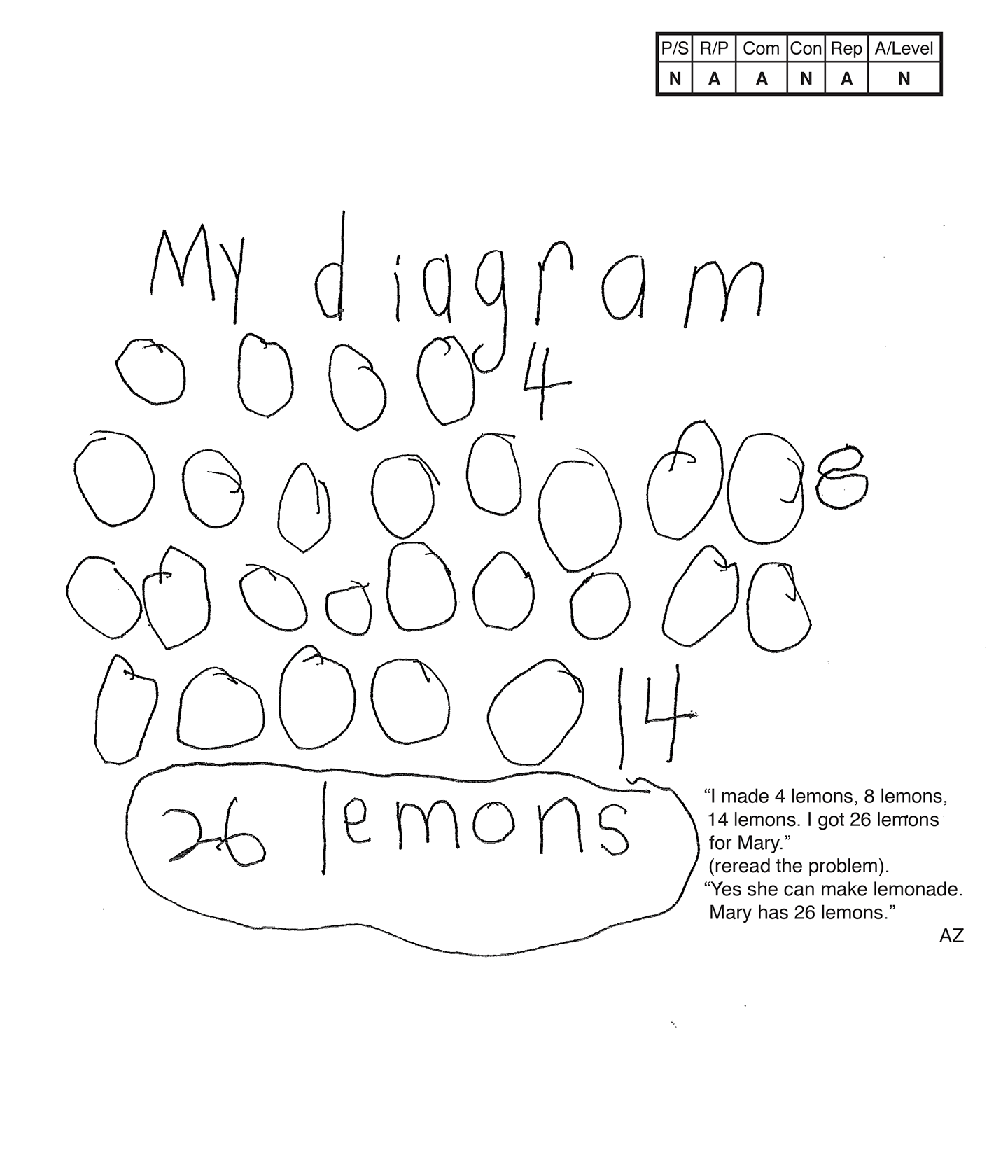This student does not meet the standard.

Scoring Rationale

Novice

The student's strategy of using a diagram to represent four, eight, and fourteen lemons, and adding all the lemons, does not work to solve the task. The student's answer, "26 lemons," is not correct.

Apprentice

The student shows some correct reasoning of the underlying concepts of the task. The student demonstrates understanding of four lemons to one glass, eight lemons to one glass. The student does not understand that the sum of four and eight lemons should be compared to fourteen lemons.

Apprentice

The student correctly uses the mathematical term diagram.

Novice

The student does not make a mathematically relevant observation.

Apprentice

The student's diagram is appropriate to part of the task but is not accurate. The student diagrams an additional fourteen lemons, which does not support a correct answer. The student defines the lemons in the scribing.

Novice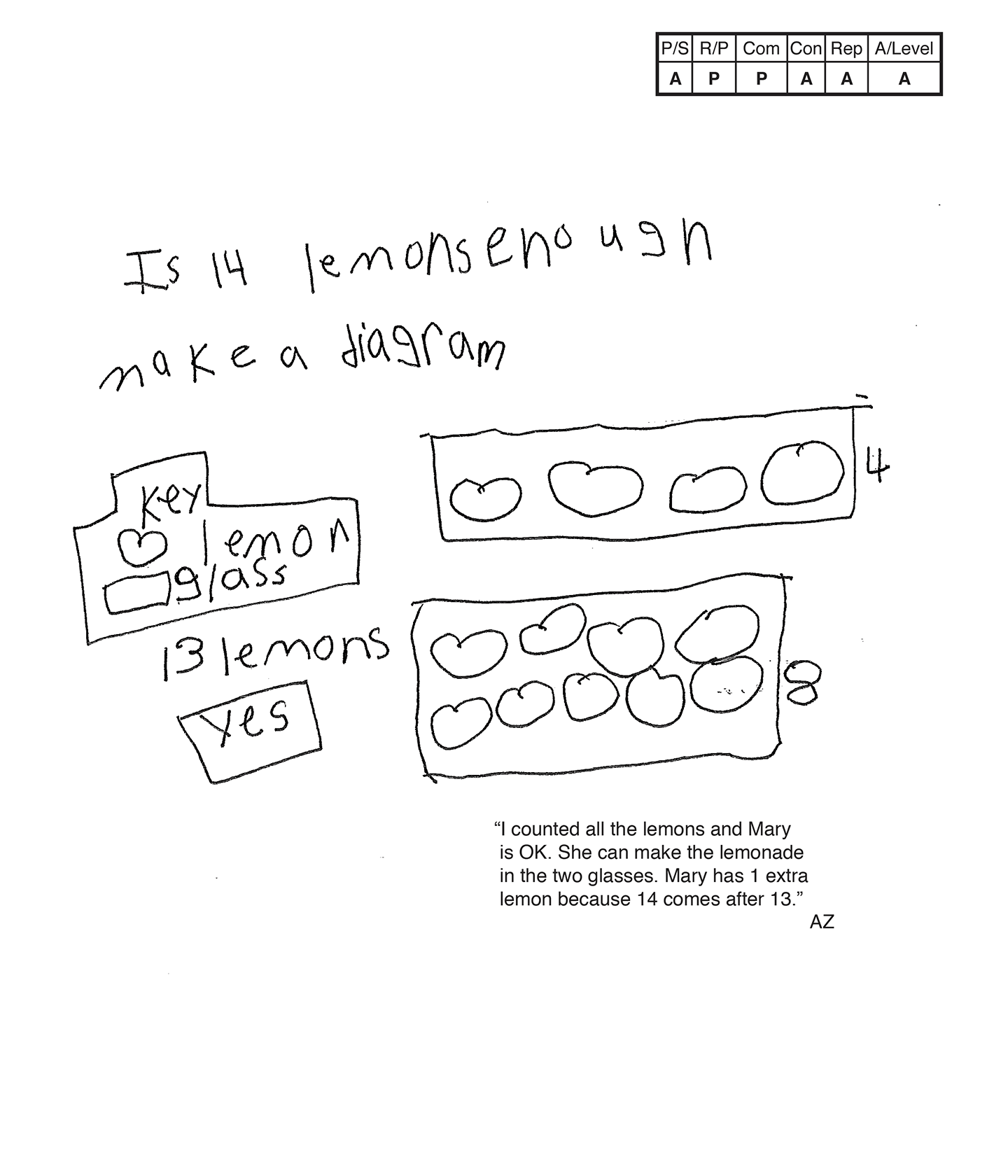This student does not meet the standard.

Scoring Rationale

Apprentice

The student's strategy of using a diagram to record how many lemons are needed to make a small and a large glass of lemonade, finding a total of lemons and comparing that total to Mary's fourteen lemons, would work to solve the task. But, the student diagrams an extra lemon in the large glass of lemonade. The student's answer, "Yes," is not correct because it is based on incorrect data.

Practitioner

The student shows correct reasoning of the underlying concepts of the task. The student demonstrates understanding of four lemons to one glass, eight lemons to one glass, finding the total number of lemons needed, and comparing that total to fourteen lemons. The student diagrams nine lemons in the large glass which leads to an incorrect total of thirteen lemons to compare to Mary's total of fourteen lemons. This is considered a careless error and not a flaw in the student's reasoning.

Practitioner

The student correctly uses the mathematical terms diagram and key.

Apprentice

The student attempts to make a mathematically relevant observation, but it is based on an incorrect diagram. The student states, "Mary has one extra lemon because fourteen comes after thirteen." The correct connection is two extra lemons.

Apprentice

The student's diagram is appropriate to the task, but is not accurate. The student diagrams an extra lemon in the large glass. A key defines the lemons and glasses.

Apprentice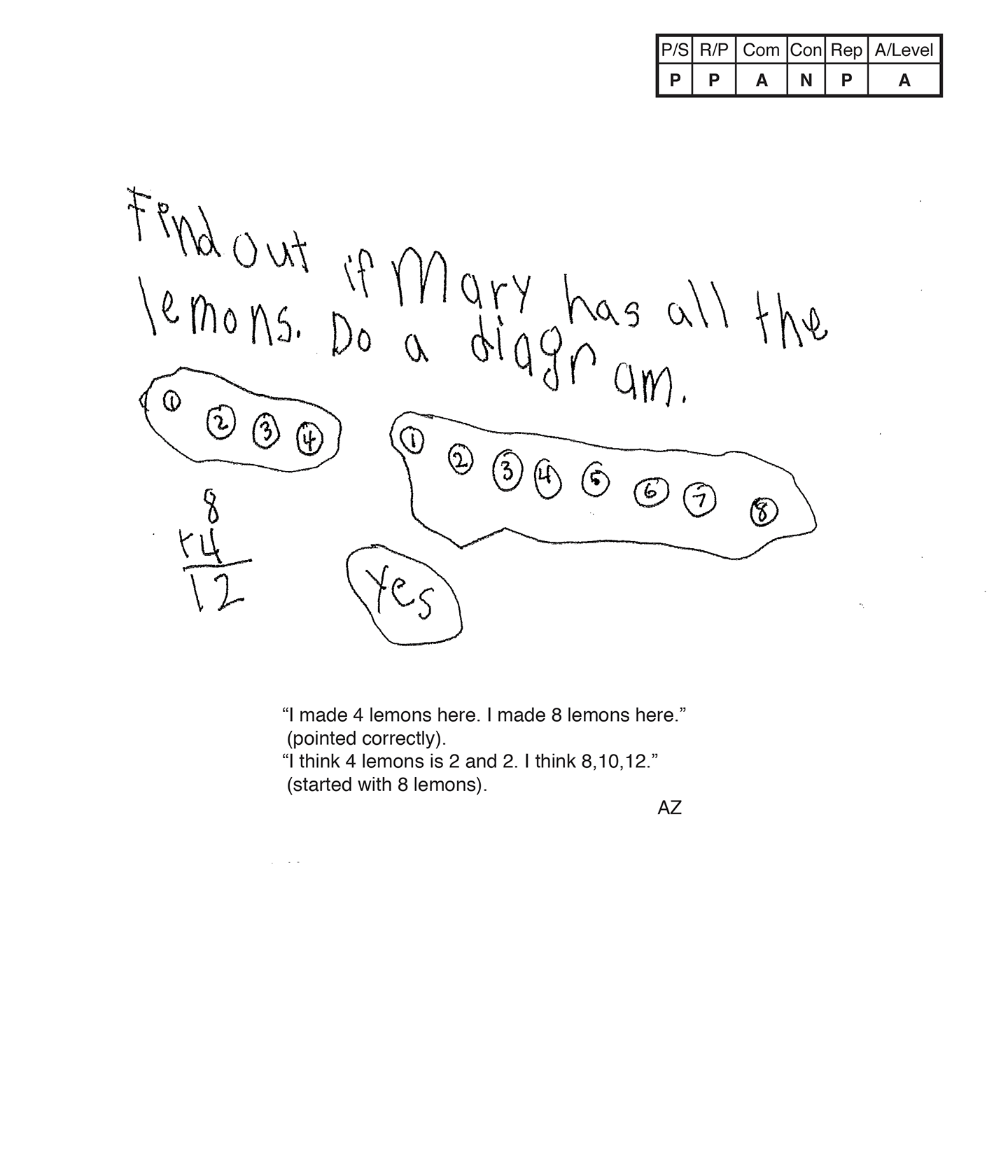This student does not meet the standard.

Scoring Rationale

Practitioner

The student's strategy of using a diagram to record how many lemons are needed to make a small and a large glass of lemonade, computing a total of twelve lemons, and comparing that total to fourteen lemons works to solve the task. The student's answer, "Yes," is correct.

Practitioner

The student shows correct reasoning of the underlying concepts of the task. The student demonstrates understanding of four lemons to one glass, eight lemons to one glass, addition to find the total number of lemons needed, and comparing that total to fourteen lemons.

Apprentice

The student correctly uses the mathematical term diagram.

Novice

The student does not make a mathematically relevant observation about their solution.

Practitioner

The student's diagram is appropriate to the task and accurate. The student's text and scribing defines the lemons.

Apprentice

Note:

The overall achievement level for this piece of student work falls under Exemplars exception to the rule category. If a student has all Apprentice scores or above, but a Novice in "Connections," the student may still receive an achievement level score of Apprentice. To learn more about Exemplars scoring, please refer to the section of your dashboard called "Tools for Success" and click on the link for “Using the Assessment Rubric.”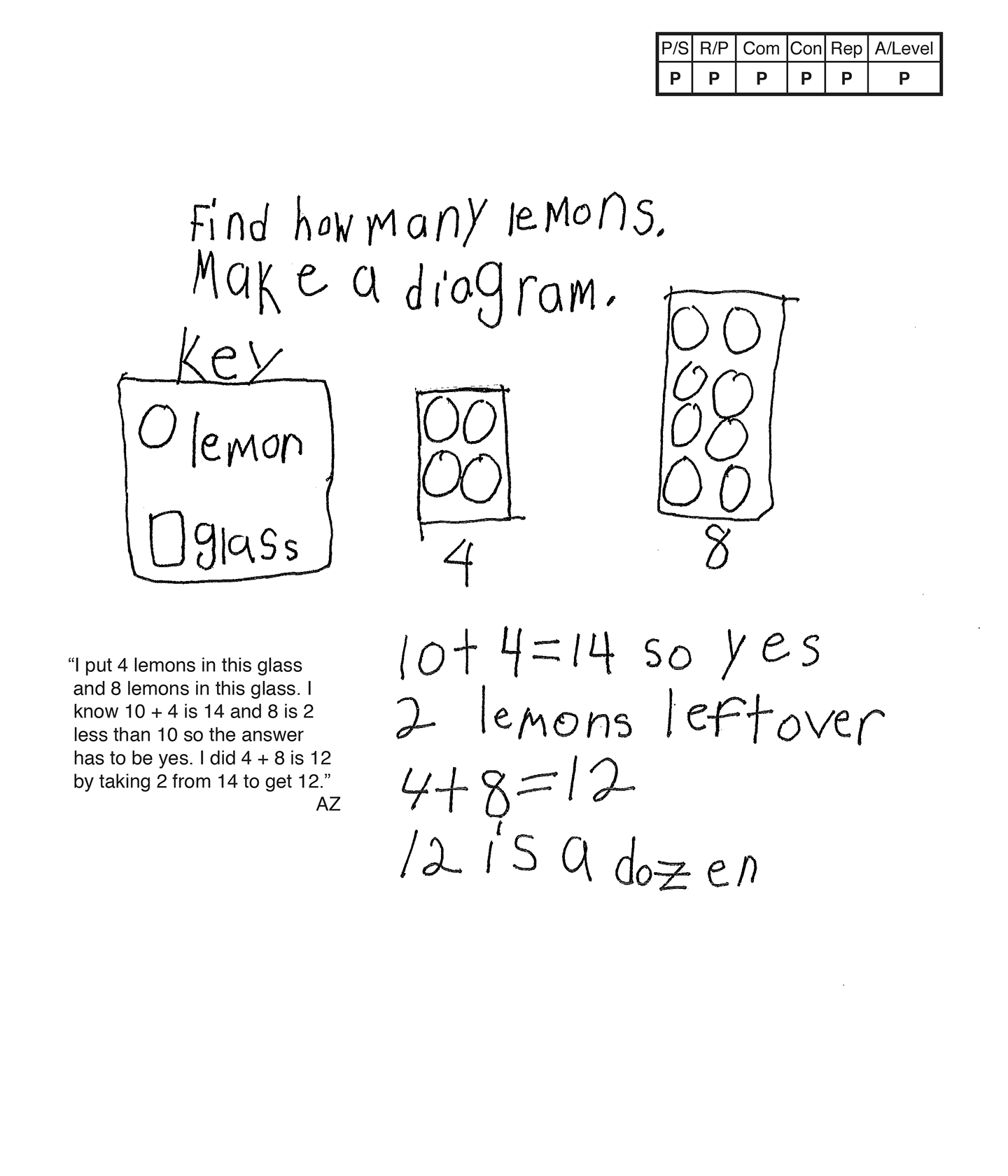This student meets the standard.

Scoring Rationale

Practitioner

The student's strategy of using a diagram to record how many lemons are needed to make a small and large glass of lemonade, and if Mary has enough lemons works to solve the task. The student's answer, "so Yes," is correct.

Practitioner

The student shows correct reasoning of the underlying concepts of the task. The student demonstrates understanding of four lemons to one glass, eight lemons to one glass, using addition to find the total number of lemons needed, and comparing that total to fourteen lemons.

Practitioner

The student correctly uses the mathematical terms diagram, key, dozen.

Practitioner

The student makes the mathematically relevant observations, "2 lemons left over," and, "12 is a dozen."

Practitioner

The student's diagram is appropriate to the task and accurate. A key defines the lemons and glasses.

Practitioner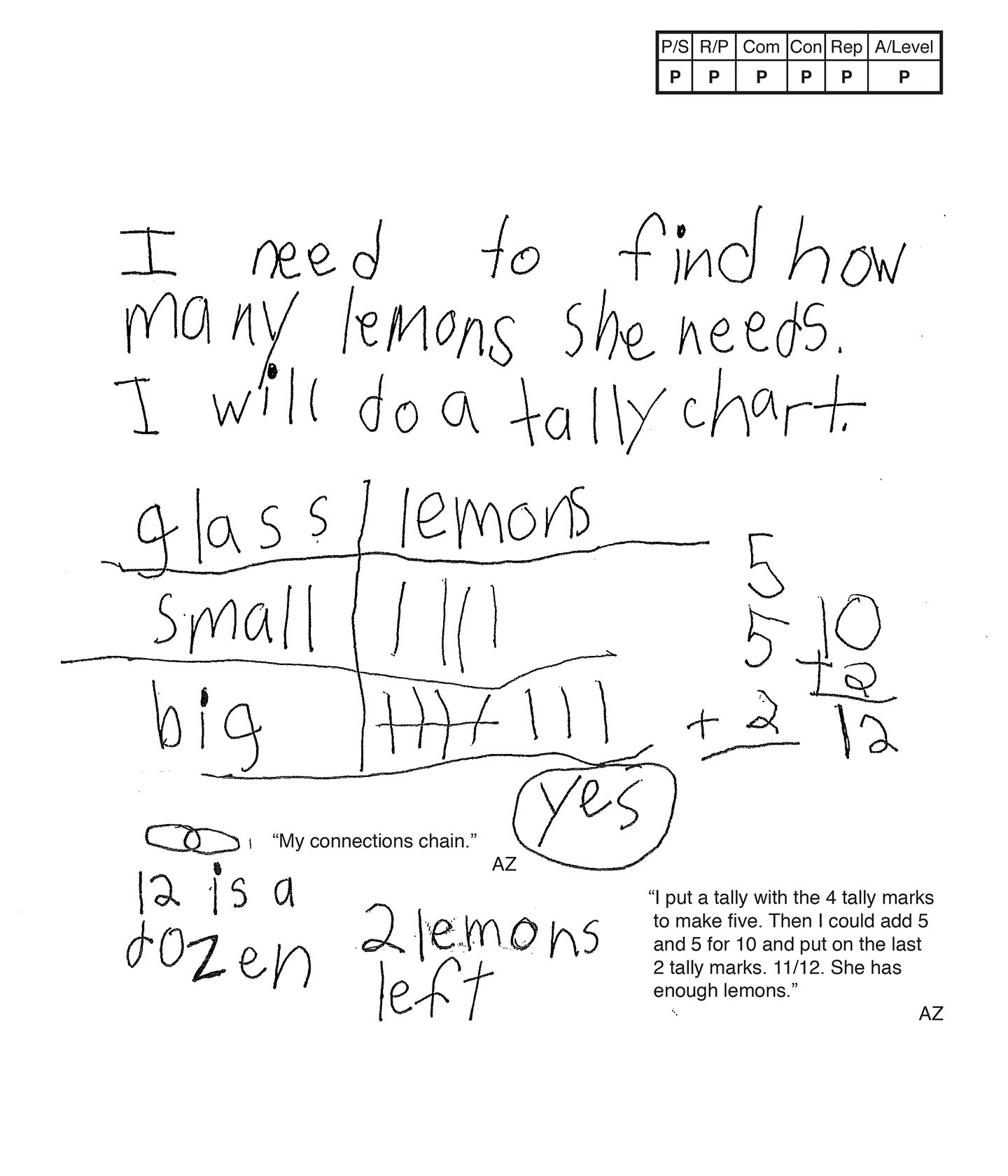This student meets the standard.

Scoring Rationale

Practitioner

The student's strategy of using a tally chart to record how many lemons are needed to make a small and large glass of lemonade, and comparing that total to Mary's fourteen lemons works to solve the task. The student's answer, "Yes," is correct.

Practitioner

The student shows correct reasoning of the underlying concepts of the task. The student demonstrates understanding of four lemons to one glass, eight lemons to one glass, using addition to find the total number of lemons needed, and comparing that total to fourteen lemons.

Practitioner

The student correctly uses the mathematical term small from the task. The student also correctly uses the terms tally chart, dozen, tally mark.

Practitioner

The student makes the mathematically relevant observations, "12 is a dozen," and, "2 lemons left."

Practitioner

The student's tally chart is appropriate to the task and accurate. All labels are provided and the data is correct.

Note: A key is not necessary if a tally mark represents one.

Practitioner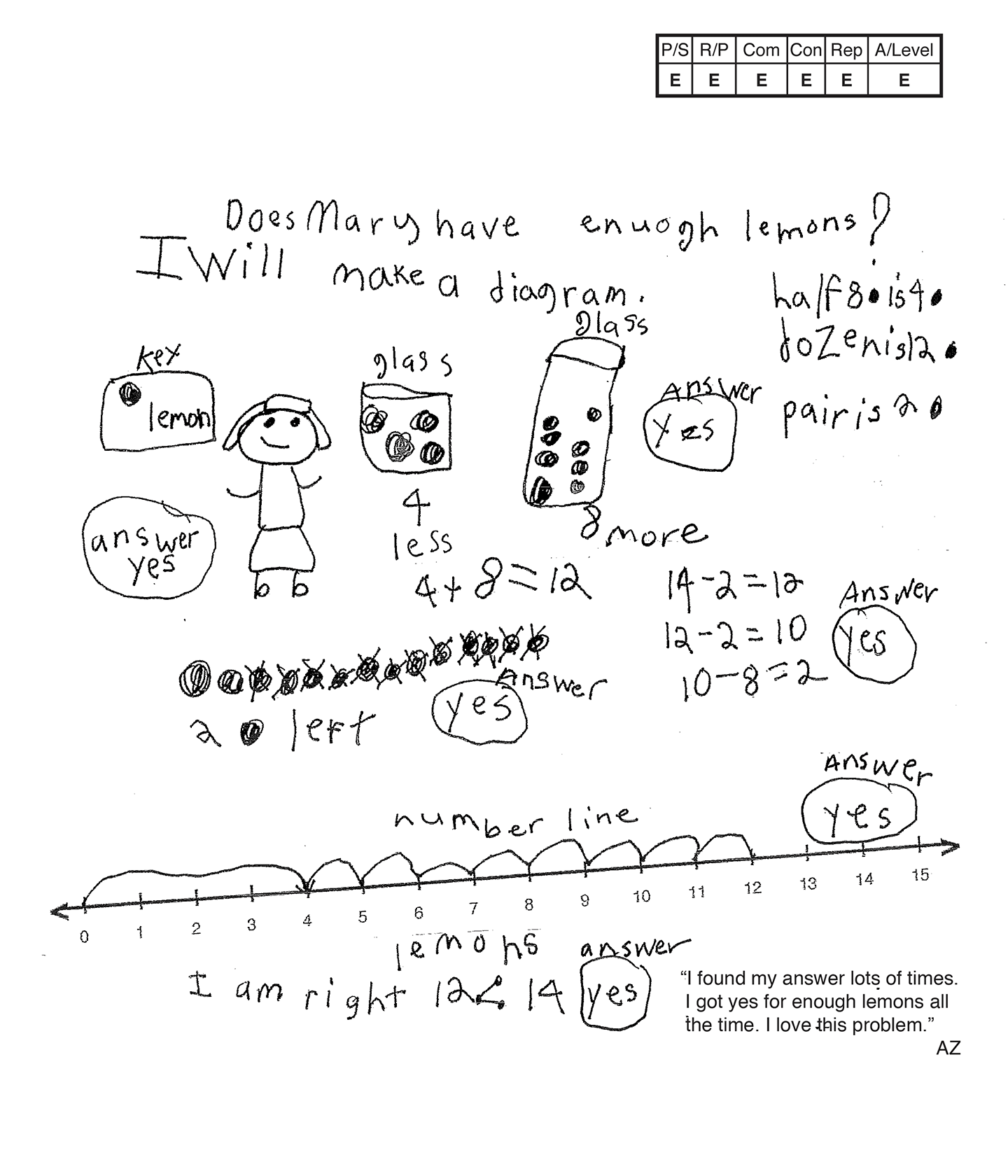This student exceeds the standard.

Scoring Rationale

Expert

The student's strategy of using a diagram of four lemons for a small glass and eight lemons for a large glass to find a total of twelve lemons to compare to fourteen lemons works to solve the task. The student's answer, "Yes," is correct. The student verifies their answer by using more strategies. The student also brings the concept of half to the problem.

Expert

The student shows correct reasoning of the underlying concepts of the task. The student demonstrates understanding of four lemons to one glass, eight lemons to one glass, finding the total number of lemons needed using addition, and comparing that total to fourteen lemons. The student uses conceptual understanding of subtraction and less than to verify their answer is correct.

Expert

The student correctly uses the mathematical terms diagram, key, half, dozen, pair, more, less, number line. The student correctly uses the mathematical notation "<".

Expert

The student makes the mathematically relevant Practitioner observations, "dozen is 12," "pair is 2 [lemons]," "4-more," "8-less," and, "2 [lemons] left." The student makes the Expert observations, "half 8 [lemons] is 4 [lemons]," and, "12 < 14." The student verifies their answer by using a second diagram to count back four and eight lemons, subtraction equations to show the two remaining lemons, a number line using an addition jump of four lemons and then eight individual lemons for a total of twelve lemons. The student states, "I found my answer lots of times. I got yes for enough lemons all the time." The student writes, "I am right."

Expert

The student's first and second diagrams are appropriate to the task and accurate. All labels are provided and a key defines the lemons. The student's number line is also appropriate and accurate with all labels included. The student links the representation to verify that their answer is correct.